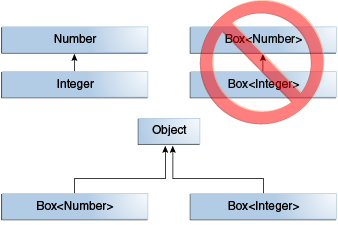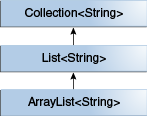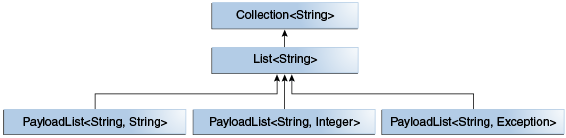Documentation

The Java™ Tutorials
Hide TOC
Trail: Learning the Java Language
Lesson: Generics (Updated)

# Generics, Inheritance, and Subtypes

As you already know, it is possible to assign an object of one type to an object of another type provided that the types are compatible. For example, you can assign an Integer to an Object, since Object is one of Integer's supertypes:

```Object someObject = new Object();
Integer someInteger = new Integer(10);
someObject = someInteger;   // OK
```

In object-oriented terminology, this is called an "is a" relationship. Since an Integer is a kind of Object, the assignment is allowed. But Integer is also a kind of Number, so the following code is valid as well:

```public void someMethod(Number n) { /* ... */ }

someMethod(new Integer(10));   // OK
someMethod(new Double(10.1));   // OK
```

The same is also true with generics. You can perform a generic type invocation, passing Number as its type argument, and any subsequent invocation of add will be allowed if the argument is compatible with Number:

```Box<Number> box = new Box<Number>();
```

Now consider the following method:

```public void boxTest(Box<Number> n) { /* ... */ }
```

What type of argument does it accept? By looking at its signature, you can see that it accepts a single argument whose type is Box<Number>. But what does that mean? Are you allowed to pass in Box<Integer> or Box<Double>, as you might expect? The answer is "no", because Box<Integer> and Box<Double> are not subtypes of Box<Number>.

This is a common misunderstanding when it comes to programming with generics, but it is an important concept to learn.Box<Integer> is not a subtype of Box<Number> even though Integer is a subtype of Number.

Note: Given two concrete types A and B (for example, Number and Integer), MyClass<A> has no relationship to MyClass<B>, regardless of whether or not A and B are related. The common parent of MyClass<A> and MyClass<B> is Object.

For information on how to create a subtype-like relationship between two generic classes when the type parameters are related, see Wildcards and Subtyping.

## Generic Classes and Subtyping

You can subtype a generic class or interface by extending or implementing it. The relationship between the type parameters of one class or interface and the type parameters of another are determined by the extends and implements clauses.

Using the Collections classes as an example, ArrayList<E> implements List<E>, and List<E> extends Collection<E>. So ArrayList<String> is a subtype of List<String>, which is a subtype of Collection<String>. So long as you do not vary the type argument, the subtyping relationship is preserved between the types.A sample Collections hierarchy

Now imagine we want to define our own list interface, PayloadList, that associates an optional value of generic type P with each element. Its declaration might look like:

```interface PayloadList<E,P> extends List<E> {
...
}
```

The following parameterizations of PayloadList are subtypes of List<String>: